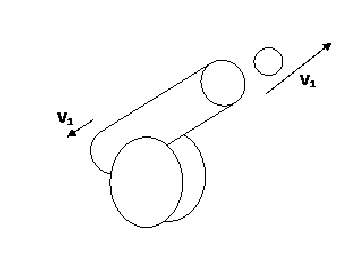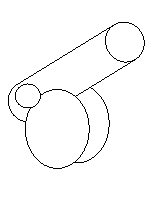Custom Search
If you want to discuss more on this or other issues related to Physics, feel free to leave a message on my Facebook page.

## Wednesday, February 10, 2010

### Principle of conservation of linear momentum

The linear momentum is the product of the mass of an object and the velocity of an object.

Now if you have two objects that are moving toward each other or one object explodes into two different objects at all time the sum of the linear momentum of the objects will give a constant number.

Hence the principle of conservation of linear momentum states that in a close system the sum of linear momentum is constant.

The equation will be that at all time

p1 +p2+p3+p4+p5+ ………= constant

Consider the diagram below:Fig 1 Before firing ballFig 2 After firing ball

As you can see from the diagram above, you have a close system that is made up of the the cannon and the cannon ball. Hence at all time the sum of the linear momentum will be a constant. Before the firing of the ball and after the firing of the ball.

Example

A canon ball is fired such that the ball leaves the cannon at a velocity of  150 m/s. If the cannon has a mass of 1000 kg and the cannon ball has a mass of 15 kg, determine the velocity of recoil of the cannon.

Hence if you apply the principle of conservation of linear momentum.

Before firing cannon ball

Sum of linear momentum before firing = pic  +pib

where pic is the initial momentum of the cannon and pib is the initial momentum of the ball.

After firing the cannon ball

sum of linear momentum after firing= pfc  +pfb

where pfc is the final momentum of the cannon and pfb is the final momentum of the cannon ball.

We are now going to determine the speed of recoil of the cannon after recoil.

Sum of linear momentum before firing = pic  +pib

= 1000*0 + 15*0

= 0 kg m/s

sum of linear momentum after firing= pfc  +pfb

=1000*vrc +15*150

=1000*vrc +2250

where is the vrc velocity or recoil of the cannon.

Hence if the sum of linear momentum is a constant

then the sum of linear momentum before firing = sum of linear momentum after firing

0 = 1000*vrc +2250

0 – 2250 = 1000*vrc

-2250 = 1000*vrc

vrc = –2.25 m/s

This principles is used also when collisions are considered. We shall see collisions in a future post.

1.2.3.